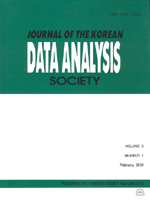상세검색
최근 검색어 전체 삭제
다국어입력
즐겨찾기0KCI등재 학술저널

# Some Structural Aspects of Ridge Partial Least Squares Regression

• 등재여부 : KCI등재
• 2014.12
• 2919 - 2930 (12 pages)

Ridge partial least squares regression (R-PLSR) is a regression method combining ridge regression and partial least squares regression and is intended to provide better predictive performance and less sensitivity to overfitting. This special regression technique was originally proposed by Kim (2007a) in a rather heuristic way. In this paper, some matrix properties and structural aspects of R-PLSR are explored in the general case where we cannot assume full-column rank of X. An alternative expression for R-PLSR is provided and the equivalence of the two forms is proved, and it is shown that the regression vector can be interpreted as the generalized inverse solution of the transformed X. Two algorithms for R-PLSR are provided and their numerical stability is investigated.

1. Introduction

2. Alternative Expression for R-PLSR

3. Interpretation of R-PLSR Solution via Generalized Inverse

4. Algorithms for R-PLSR

5. Numerical Examples